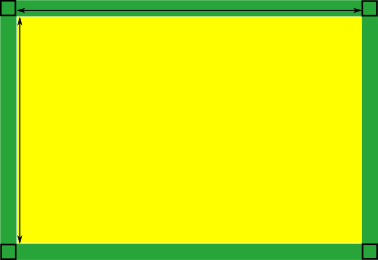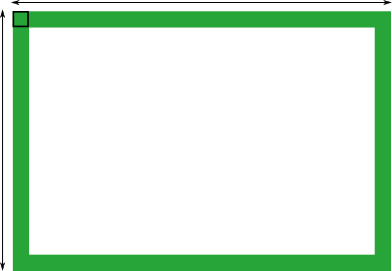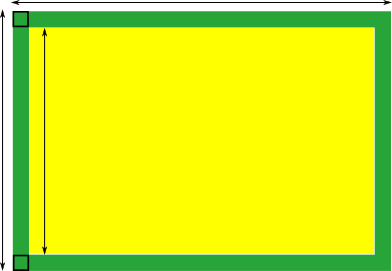You may also likeHallway Borders

What are the possible dimensions of a rectangular hallway if the number of tiles around the perimeter is exactly half the total number of tiles?Square Pegs

Which is a better fit, a square peg in a round hole or a round peg in a square hole?Boxed In

A box has faces with areas 3, 12 and 25 square centimetres. What is the volume of the box?

Tile Border

Age 11 to 14 ShortChallenge Level

Rectangles that look most like squares will have the largest areas relative to their perimeters.

Considering the length and width of the yellow rectangle
Looking at the perimeter of the yellow rectangle, the 4 green tiles at the corners will not be included, as shown below.So if there are 62 green tiles altogether, then 58 of them will touch the yellow rectangle. So the perimeter of the yellow rectangle is 58 tiles.

This means that the length and width of the yellow rectangle add up to half of 58 - which is 29.

To make a rectangle with the largest possible area, we should choose lengths to make it like a square - so 14 by 15 will work, since 14 + 15 = 29. So the yellow rectangle will be made of 14 $\times$ 15 $=$ 210 yellow tiles.

Making the perimeter of the 'largest' possible rectangle using the green tiles
Just imagining the 62 tiles that form the perimeter, we can work out how large the length and width of the whole rectangle can be.If we worked out the distance around the outide of the green rectangle, then the 4 corner tiles will all be counted twice. So if there are 62 green tiles, the perimeter of the whole rectangle is 66.

That means that the length added to the width of the whole rectangle is 33.

To make a rectangle with the largest possible area, we should choose lengths to make it like a square - so 16 by 17 will work, since 16 + 17 = 33.

That means that the yellow rectangle on the inside will be 14 by 15 (there are geen tiles above and below, and left and right, as shown below, so we need to subtract 2).So the yellow rectangle will be made of 14 $\times$ 15 $=$ 210 yellow tiles.

You can find more short problems, arranged by curriculum topic, in our short problems collection.